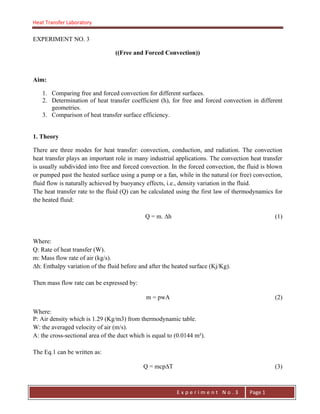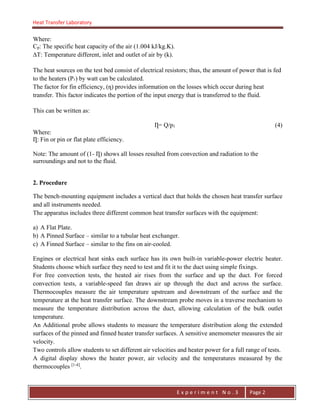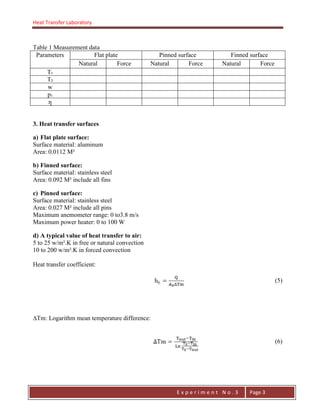Se está descargando tu SlideShare. ×

# Free and Forced Convection

Anuncio
Anuncio
Anuncio
Anuncio
Anuncio
Anuncio
Anuncio
Anuncio
Anuncio
Anuncio
AnuncioAnuncio
Cargando en…3
×

1 de 5 Anuncio

# Free and Forced Convection

There are three modes for heat transfer: convection, conduction, and radiation. The convection heat transfer plays an important role in many industrial applications. The convection heat transfer is usually subdivided into free and forced convection. In the forced convection, the fluid is blown or pumped past the heated surface using a pump or a fan, while in the natural (or free) convection, fluid flow is naturally achieved by buoyancy effects, i.e., density variation in the fluid.

There are three modes for heat transfer: convection, conduction, and radiation. The convection heat transfer plays an important role in many industrial applications. The convection heat transfer is usually subdivided into free and forced convection. In the forced convection, the fluid is blown or pumped past the heated surface using a pump or a fan, while in the natural (or free) convection, fluid flow is naturally achieved by buoyancy effects, i.e., density variation in the fluid.

Anuncio
Anuncio

### Free and Forced Convection

1. 1. Heat Transfer Laboratory E n g . H a y m e n F . F a t t a h E x p e r i m e n t N o . 3 Page 1 EXPERIMENT NO. 3 ((Free and Forced Convection)) Aim: 1. Comparing free and forced convection for different surfaces. 2. Determination of heat transfer coefficient (h), for free and forced convection in different geometries. 3. Comparison of heat transfer surface efficiency. 1. Theory There are three modes for heat transfer: convection, conduction, and radiation. The convection heat transfer plays an important role in many industrial applications. The convection heat transfer is usually subdivided into free and forced convection. In the forced convection, the fluid is blown or pumped past the heated surface using a pump or a fan, while in the natural (or free) convection, fluid flow is naturally achieved by buoyancy effects, i.e., density variation in the fluid. The heat transfer rate to the fluid (Q) can be calculated using the first law of thermodynamics for the heated fluid: Q = m. ∆h (1) Where: Q: Rate of heat transfer (W). m: Mass flow rate of air (kg/s). ∆h: Enthalpy variation of the fluid before and after the heated surface (Kj/Kg). Then mass flow rate can be expressed by: m = pwA (2) Where: P: Air density which is 1.29 (Kg/m3) from thermodynamic table. W: the averaged velocity of air (m/s). A: the cross-sectional area of the duct which is equal to (0.0144 m²). The Eq.1 can be written as: Q = mcp∆T (3)
2. 2. Heat Transfer Laboratory E n g . H a y m e n F . F a t t a h E x p e r i m e n t N o . 3 Page 2 Where: Cp: The specific heat capacity of the air (1.004 kJ/kg.K). ∆T: Temperature different, inlet and outlet of air by (k). The heat sources on the test bed consist of electrical resistors; thus, the amount of power that is fed to the heaters (P₁) by watt can be calculated. The factor for fin efficiency, (ƞ) provides information on the losses which occur during heat transfer. This factor indicates the portion of the input energy that is transferred to the fluid. This can be written as: Ƞ= Q/p1 (4) Where: Ƞ: Fin or pin or flat plate efficiency. Note: The amount of (1- Ƞ) shows all losses resulted from convection and radiation to the surroundings and not to the fluid. 2. Procedure The bench-mounting equipment includes a vertical duct that holds the chosen heat transfer surface and all instruments needed. The apparatus includes three different common heat transfer surfaces with the equipment: a) A Flat Plate. b) A Pinned Surface – similar to a tubular heat exchanger. c) A Finned Surface – similar to the fins on air-cooled. Engines or electrical heat sinks each surface has its own built-in variable-power electric heater. Students choose which surface they need to test and fit it to the duct using simple fixings. For free convection tests, the heated air rises from the surface and up the duct. For forced convection tests, a variable-speed fan draws air up through the duct and across the surface. Thermocouples measure the air temperature upstream and downstream of the surface and the temperature at the heat transfer surface. The downstream probe moves in a traverse mechanism to measure the temperature distribution across the duct, allowing calculation of the bulk outlet temperature. An Additional probe allows students to measure the temperature distribution along the extended surfaces of the pinned and finned heater transfer surfaces. A sensitive anemometer measures the air velocity. Two controls allow students to set different air velocities and heater power for a full range of tests. A digital display shows the heater power, air velocity and the temperatures measured by the thermocouples [1-4] .
3. 3. Heat Transfer Laboratory E n g . H a y m e n F . F a t t a h E x p e r i m e n t N o . 3 Page 3 Table 1 Measurement data Parameters Flat plate Pinned surface Finned surface Natural Force Natural Force Natural Force T₁ T₂ w p₁ ƞ 3. Heat transfer surfaces a) Flat plate surface: Surface material: aluminum Area: 0.0112 M² b) Finned surface: Surface material: stainless steel Area: 0.092 M² include all fins c) Pinned surface: Surface material: stainless steel Area: 0.027 M² include all pins Maximum anemometer range: 0 to3.8 m/s Maximum power heater: 0 to 100 W d) A typical value of heat transfer to air: 5 to 25 w/m².K in free or natural convection 10 to 200 w/m².K in forced convection Heat transfer coefficient: (5) ∆Tm: Logarithm mean temperature difference: (6)
4. 4. Heat Transfer Laboratory E n g . H a y m e n F . F a t t a h E x p e r i m e n t N o . 3 Page 4 Fig.1 Free and forced convection apparatus with screenshot of the optional VDAS® Software  . 4. Discussion questions a) Why we get different heat transfer coefficient (h) for the same material? b) Why we have errors? c) Discuss the (hc-Ƞ) diagram that you draw it. d) What do you suggest to get a better reading? References 1. TecQuipment Ltd, Free and forced convection apparatus, Model: VDAS TD1005. 2. Мохамед Б, Кароли Я, Зеленцов А.А. (2020) Трехмерное моделирование течения газа во впускной системе автомобиля «формулы студент» Журнал Сибирского федерального университета, 13(5); pp. 597-610. https://doi.org/10.17516/1999-494X- 0249.
5. 5. Heat Transfer Laboratory E n g . H a y m e n F . F a t t a h E x p e r i m e n t N o . 3 Page 5 3. Mohamad B., Karoly J., Zelentsov A.A. (2020) Hangtompító akusztikai tervezése hibrid módszerrel, Multidiszciplináris Tudományok, 9(4), pp. 548-555. https://doi.org/10.35925/j.multi.2019.4.58. 4. Yunus Cengel, Heat Transfer: A Practical Approach, 2nd ed., McGraw-Hill Education – Europe, 1997. Barhm Abdullah Mohamad Erbil Polytechnic University LinkedIn: https://www.linkedin.com/in/barhm-mohamad-900b1b138/ Google Scholar: https://scholar.google.com/citations?user=KRQ96qgAAAAJ&hl=en ResearchGate: https://www.researchgate.net/profile/Barhm_Mohamad YouTube channel: https://www.youtube.com/channel/UC16-u0i4mxe6TmAUQH0kmNw